# Math Symbols Worksheets

#### What are Symbols Used for In Math?

Mathematical symbols can be difficult and can form a barrier to learning mathematical concepts. Now, different symbols are used in mathematics and hold significant meaning as well. Let us list down a few of those symbols for you.

 Operation Symbol addition + subtraction - multiplication x, * division ÷, / grouping values [ ], ( ) sets { } pi π infinity ∞ equal to = approximately equal ≅ not equal to ≠ less than <, ≤ greater than >, ≥ radical symbol √ degrees °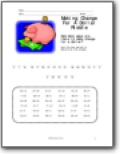###### Making Change For A Dollar Riddle

How many ways are there to make change for a dollar? Use the key below to determine the answer.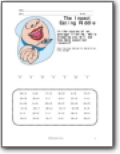###### Eating Insects

Use the key to determine how many insects you will eat in your lifetime. Not on purpose...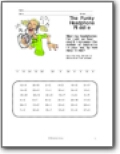Wearing headphones for just an hour could increase the number of bacteria in your ear by how many times?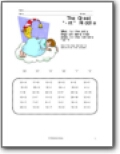###### The Great "-mt" Riddle

What is the only English word that ends in the letters "mt"?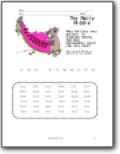###### The Ratty Riddle

Rats multiply very quickly. In eighteen months, how many descendants could two rats have?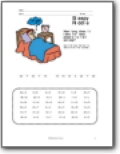###### Sleepy Riddle

How long does it take for most people to fall asleep?